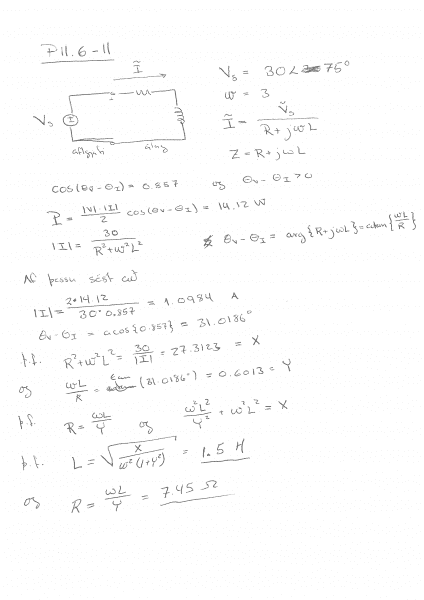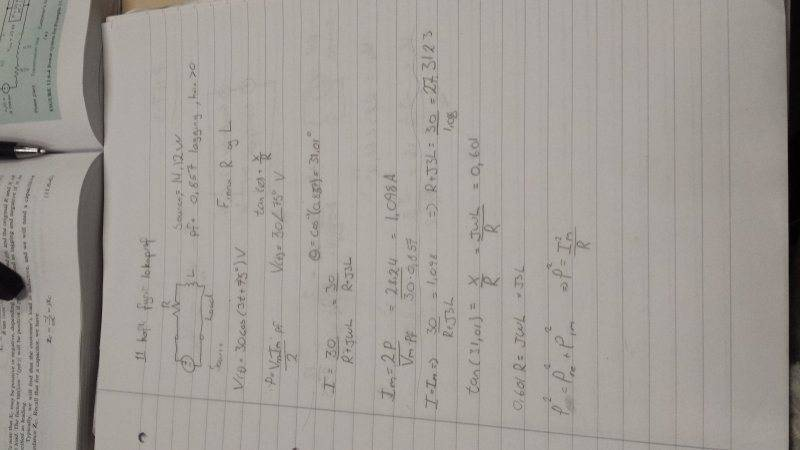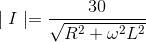# Basic RL circuit -- Power example

• Engineering

## Homework Statement

A simple RL circuit in series connected to a source with V(t) = 30Cos(3t+75°)V and is delivering 14.12 W of power. I'm also given the power factor which is pf=0.857 and is lagging.

Find the values of R and L

## Homework Equations

P=Vm*Im/2 * pf for power
theta = Cos^-1(pf)

## The Attempt at a Solution

I've tried the problem and I already have the solution which I will link to this post. But I do not understand some part of the problem.
So what I've basically done is I found the angle of the power factor by using the equation above.
Theta = Cos^-1(0.857) = 31.01° ( positive because the powerfactor is lagging)

Then by Ohms law I find the Current which is I = V/Z and Z = R + jwl
so I = V/(R + jwl). We know that the Voltage is sinusoidal and therefor Vm = 30 V .
Then we have I = 30/(R +jwl)

I can also find maximum current by using the eqation stated above. Which is
P=Vm * Im / 2 * pf ----> algebra ---> Im = 2*P/(Vm*pf) = 1.098 A

This is how far I could get and I don't know how to get anything else. But in the solution I have it says that
| I | = 30/(R^2 + w^2 * L^2) .... I have absolutely no idea how this is true? But what I find the most disturbing is that I thought that the impedance of the Inductor would be "Jwl" not only "wL", Where is the "J" in that eqation?[/B]

same thing with given solution that (theta)v - (theta)i = avg{R + jwl} = atan(wl/R} .... <-- what is this. Where does this come from and where is the "J"

In the end my solution tries to find two other variables called X and Y. I assume that X is the reactance, and once again I do not know what Y stands for.
The solutions says R^2 + w^2 * L^2 = 30/| I | = 27.3123 = X <--- again where is that J and why is it not there. I need the explanation.
To find Y variable you use this equation R = wl/X and from that w^2 * L^2 / Y ^2 + w^2 L^2 = X
I do not understand what was happening there.

So to sum it all up. I am confused why there is no 'J' in some of the equations and why they are squared in others.
It would be greatly appreciated if I could get some explanation on how this power transfer works.

I will link to pictures with this post. One with my attempt and one of the solution.But in the solution I have it says that
| I | = 30/(R^2 + w^2 * L^2) .... I have absolutely no idea how this is true?
It is not true. Correct formula is:Notice also this is a peak current since peak voltage is 30 V.

It is not true. Correct formula is:Notice also this is a peak current since peak voltage is 30 V.

Alright thanks for that, but isn't the impedance for inductor Zl = Jwl?

Alright thanks for that, but isn't the impedance for inductor Zl = Jwl?
It is. Total impedance is Z=R+jωL, and it's modulus IZI2=R22L2

It is. Total impedance is Z=R+jωL, and it's modulus IZI2=R22L2

Thank you very much. Problem solved. I just couldn't figure out what the solution was telling me but once you said they were wrong It all came together.

Thanks again for the help :D